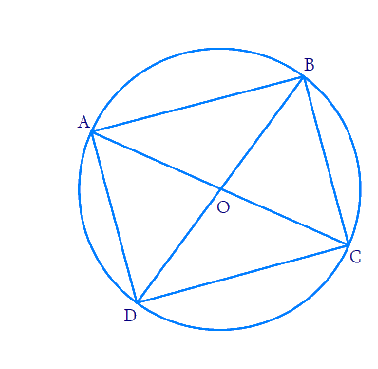In the verge of coronavirus pandemic, we are providing FREE access to our entire Online Curriculum to ensure Learning Doesn't STOP!

# Ex.10.5 Q7 Circles Solution - NCERT Maths Class 9

Go back to  'Ex.10.5'

## Question

If diagonals of a cyclic quadrilateral are diameters of the circle through the vertices of the quadrilateral, prove that it is a rectangle.

Video Solution
Circles
Ex 10.5 | Question 7

## Text Solution

What is known?

Diagonals of a cyclic quadrilateral are diameters of a circle passing through the vertices.

What is unknown?

To prove lines joining the vertices is a rectangle.

Reasoning:

• The angle subtended by an arc at the centre is double the angle subtended by it at any point on the remaining part of the circle.
• The sum of either pair of opposite angles of a cyclic quadrilateral is $$180^{\circ}.$$
• Diameter is a chord.

Steps:Let $${DB}$$ be the diameter of the circle which is also a chord.

Then  \begin {align} \angle {BOD}=180^{\circ} \end {align}

We know that, the angle subtended by an arc at the centre is double the angle subtended by it at any point on the remaining part of the circle.

\begin{align} \angle {BAD} &=\frac{1}{2} \times \angle {BOD} \\ &=90^{\circ} \end{align}

Similarly,  \begin {align} \angle {BCD}=90^{\circ} \end {align}

Now considering $$\text{AC}$$ as the diameter of the circle, we get \begin {align} \angle {AOC}=180^{\circ} \end {align}

We know that, the angle subtended by an arc at the centre is double the angle subtended by it at any point on the remaining part of the circle.

\begin{align} \angle {ABC} &=\frac{1}{2} \times \angle {AOC} \\ &=90^{\circ} \end{align}

Similarly, \begin {align} \angle {ADC}=90^{\circ} \end {align}

As you can see, all the angles at the corners are $$90^\circ$$we can say that the shape joining the vertices is a rectangle.

This problem can also be solved by using the property of cyclic quadrilaterals.

Video Solution
Circles
Ex 10.5 | Question 7

Learn from the best math teachers and top your exams

• Live one on one classroom and doubt clearing
• Practice worksheets in and after class for conceptual clarity
• Personalized curriculum to keep up with school